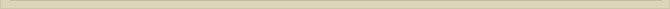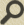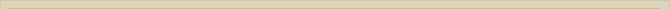## The electric field and the ether

When you are interested in physics you must read “Unbelievable“!

First we describe the physical properties the ether should have to be consistent with the vacuum and the observed electrostatic field. We follow a path unknown and different in many ways from generally accepted theories leading to other thoughts and possibilities so diverged that the consequences induces strong rejection established science.

Objectively the described ether only has to be consistent with observations. The criticism against ether is determined current acknowledged theses and not fundamental objections. The ether theory asks for an unbiased attitude, because many perspectives are new and contrary to the present perceptions of science. It is easy to just ridicule the ether theory, but science should know better. Scientists should always be open to new ideas. Arguments should be directive to the outcome of the debate and not prejudice. No thought should be denied without reason.

If there is ether, than ether hides in a vacuum. The speed of light c, almost 300,000 km/s, is one of the characteristics of vacuum and therefore of the ether. The electric characteristic of vacuum is determined  εo while the magnetic properties are described  μo. The relation between the electric and magnetic properties of vacuum is given :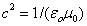The speed of light c, however high it may seem, can be considered as the inert quality of vacuum respectively the lag of ether to transfer electrostatic changes through space in time. When there are electrostatic changes it takes time to adjust for vacuum respectively the ether surrounding the source. The time lag to transfer electrostatic changes in space is given the speed of light c.

Electric charges in space can be held responsible for a dielectric shift in the ether. The result of the dielectric displacement is a static electric field. Electric charges polarize ether. The natural constant in vacuum for this physical phenomenon is the dielectric susceptibility εo. When we place a charge Q in space at t=0 the surrounding ether will be polarized with the speed of light cεo tells us something about the force needed to attain a shift of electric charge in space. The strength of an electric field is in physics given :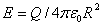The equation indicates that the influence of charge Q on the ether declines when the distance to Q increases. The blast of an explosion diminishes in a same way when the distance changes. E, in the equation, is than the loudness of the blast at distance R. The electric field around Q builds up with the velocity c (the blast with the speed of sound). With ether the process of shifting charges in space can be described.

When we assume an ether is present in vaccuum than the ether should be the medium where the electric field originates resp. exists. In ether there has to be positive and negative charge elements to be able to explain the electric field. If we assume ether is some sort of perfect fluid where positive- and negative charge elements are present and can separate and an electric field occurs, we encounter an inconsistency in explaining the electromagnetic properties of vacuum.

When there is free positive and negative charge in ether a charge in vacuum would instantly be shielded the opposite charge in the fluidic ether. An electric field then not exist. So to be able to describe the electric field we have to assume that the ether is confined to an identity. The positive and negative charge in ether cannot be totally free.

The natural constants εo, μo and c describes the physics of vacuum and therefore the ether it contains. The polarization process can be understood assuming the ether, vacuum, existing of very small neutral point-volumes. The assumption of an identity like the point-volume is necessary to be able to describe the physics concerning the electrostatic field. The assumption of the existence of point-volumes may seem remarkable at first.

We further assume each neutral point-volume is the result of two overlapping point-volumes of opposite sign; a negative and a positive point-volume. The negative and positive point-volume can be (partly) separated depending on the strength of the electric field. The vacuum is considered to be filled with point-volumes.

These assumptions do not add any physical characteristics to the vacuum we already know. The entities ε, μ and c already implies these now visualized characteristics. The assumption of point-volumes is just the means to visualize the process. When there is an electric field the strength of this field is considered reciprocally proportional with the squared distance of the positive and negative point-volume. Schematically the separation is demonstrated in figure 8.

Compare the process with a very small and very strong spring. When there is an electric field E on opposite sides of the point-volume, there are equal forces pulling apart the positive and negative part. When there is for example a positive charge +Q in the ether, then in the point-volumes next to +Q the negative part of the point-volume is attracted and the positive is rejected. The separation in these point-volumes initiates a separation, in a similar way, in the next point-volume and so on. In this way one can envisage the existence of an electric field in vacuum caused an electric charge.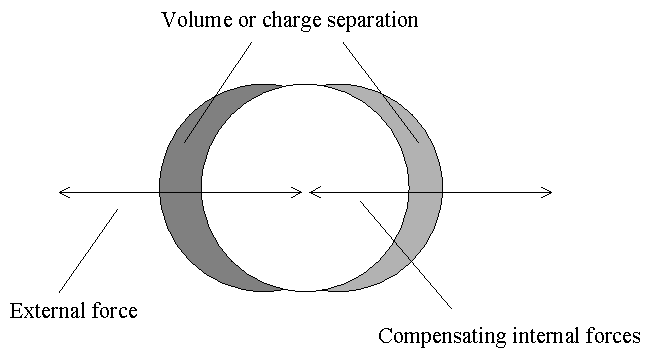Figure 8.  The charge or volume separation in a point-volume.

The charge +Q attracts charge in the ether surrounding it to the extent that and until the charge +Q is neutralized a shift –Q in the adjacent point-volumes. The charge is neutralized. The charge shift in the adjacent point-volumes is again neutralized the shift in the next point-volume etc. With the speed of light c the dielectric displacement of charge +Q occurs in space. Although there is a new balance in the ether after X/c sec at distance X from Q, there is still a tension in the ether; the electric field E.

Summarizing the previous; the charge +Q placed in the ether polarizes the ether and at the same time the polarization neutralizes the charge. The tension in the ether is caused the shift of charge in the point-volumes surrounding the charge. The tension in the ether (E) diminishes with the increase in distance R according to the formula:Before the charge +Q is placed in the ether there was no interaction between the point-volumes. There was no shift of charge in the ether respectively the point-volumes. The dielectric movement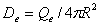is responsible for an interactive force between the point-volumes and is the cause for the force between two charges expressed the Coulomb force: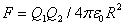The charge separation, respectively the negative and positive volume displacement in the point-volumes, cannot be free to leave the point-volume, because the original charge +Q would disappear and than no electric field E could be observed anymore. There must be a charge displacement in the point-volume or ether otherwise the charge would leave the point-volumes and annihilate the charge +Q.

In an electric field the charge shift must be restricted to the imaginary point-volumes. The smallest charge known is the charge of an electron –Qe and that of its anti-particle the positron +Qe. This is the reason why we assume the neutral point-volumes exists of overlapping negative (-Qe) and a positive point-volumes (+Qe), equal to the elementary charge of an electron.

For describing the electric field we assume ether with the well-known electromagnetic properties of vacuum, described :Logic determined we have to consider ether to be encapsulated; that the negative and positive charges cannot be totally free. We added only “visual” entities coexisting with the electromagnetic properties of vacuum. These visual images are the only means which to describe the physical process and they are completely consistent with the physical characteristics of vacuum.

As a fact no physical characteristics are added visualizing the assumed process. With ether this is possible. On the other hand the current perception of electromagnetic characteristics of vacuum has mysterious sides caused the assumed empty space and relativity of time and space.

In the perception of science according to SRT vacuum is an absolute empty space, where there is no possibility to comprehend the dielectric movement or an electric field; only mathematical formulation is possible. A light beam with the present perception (SRT) will, when it leaves the earth, reach the end of the universe immediately. The travel time of the photon is nil. The “clock” of the photon stands still because the photon travels with speed c. Measured in our time it will take billions of years for the photon to reach the end of the universe. Time and space exists with the Relativity Theory, but at the same time they do not!

The assumption of an absolute empty space theoretical allows the consequences of SRT; the relativity of time and space. The present perception of science concerning vacuum is however not anymore the original absolute empty space of SRT assumed Einstein, but one that allows electric and magnetic fields (field theory) which however cannot exist in an assumed absolute empty space! So the concept of an absolute empty space is actually already abandoned science, but the theoretical consequences of the relativity of time and space are still considered to be valid!!!

It appears Science does not dare to accept the theoretical consequences the shift in perspective concerning vacuum (SRT) and field theory actually implies. It is always hard to acknowledge mistakes. Because Theoretical Physics refuses to adjust to the altered insights it becomes more and more a religion rather than a science.

Next chapter: The Lorentzfactor and the ether